# Electronics and Communication Engineering - Automatic Control Systems

### Exercise :: Automatic Control Systems - Section 1

41.

For the control system in the given figure, the value of K for critical damping is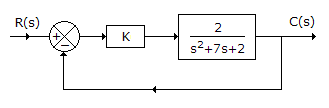A. 1 B. 5.125 C. 6.831 D. 10

Explanation: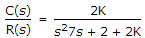since ζ = 1, ωn = 3.5 Hence 2 + 2k = 3.52 = 12.25 or K = 5.125 .

42.

Bode magnitude plot is drawn between

 A. magnitude of network function and ω B. dB magnitude and log ω C. dB magnitude and ω D. loge (magnitude) and log ω

Explanation:

Frequency is plotted on log scale and magnitude is taken in dB.

43.

For the system in the given figure,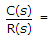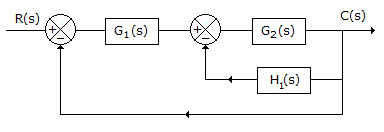A.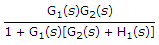B.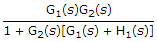C.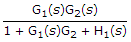D. none of the above

Explanation:

For inner loop we have transfer function =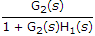Then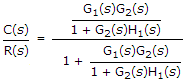=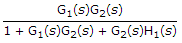.

44.

In the given figure, if R = XC, voltage gain is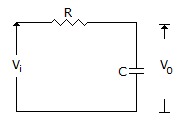A. 0 dB B. 3 dB C. -3 dB D. 20 dB

Explanation:

If R = XC, V0 = 0.5 Vi Hence gain is -3 dB.

45.

For the circuit in the given figure, V2(s)/V1(s) =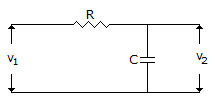A.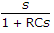B.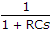C.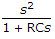D.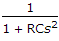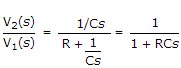.